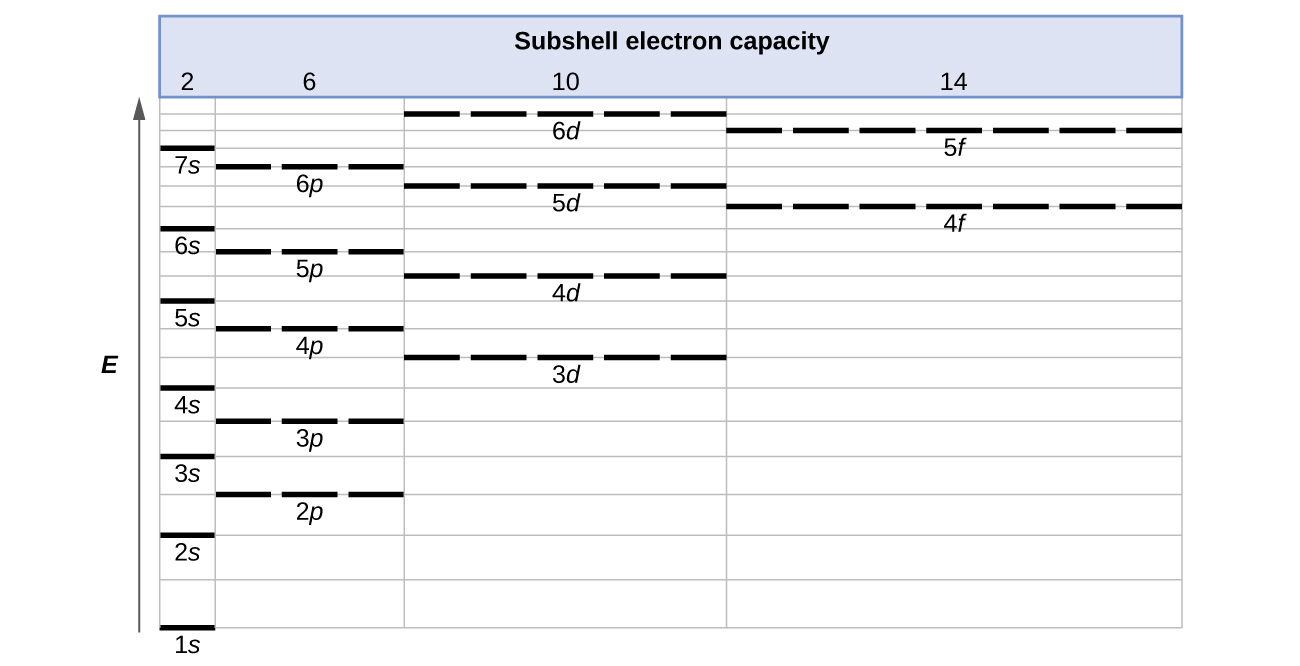# 6.4 Electronic structure of atoms (electron configurations)

 Page 1 / 15
By the end of this section, you will be able to:
• Derive the predicted ground-state electron configurations of atoms
• Identify and explain exceptions to predicted electron configurations for atoms and ions
• Relate electron configurations to element classifications in the periodic table

Having introduced the basics of atomic structure and quantum mechanics, we can use our understanding of quantum numbers to determine how atomic orbitals relate to one another. This allows us to determine which orbitals are occupied by electrons in each atom. The specific arrangement of electrons in orbitals of an atom determines many of the chemical properties of that atom.

## Orbital energies and atomic structure

The energy of atomic orbitals increases as the principal quantum number, n , increases. In any atom with two or more electrons, the repulsion between the electrons makes energies of subshells with different values of l differ so that the energy of the orbitals increases within a shell in the order s < p < d < f. [link] depicts how these two trends in increasing energy relate. The 1 s orbital at the bottom of the diagram is the orbital with electrons of lowest energy. The energy increases as we move up to the 2 s and then 2 p , 3 s , and 3 p orbitals, showing that the increasing n value has more influence on energy than the increasing l value for small atoms. However, this pattern does not hold for larger atoms. The 3 d orbital is higher in energy than the 4 s orbital. Such overlaps continue to occur frequently as we move up the chart.Generalized energy-level diagram for atomic orbitals in an atom with two or more electrons (not to scale).

Electrons in successive atoms on the periodic table tend to fill low-energy orbitals first. Thus, many students find it confusing that, for example, the 5 p orbitals fill immediately after the 4 d , and immediately before the 6 s . The filling order is based on observed experimental results, and has been confirmed by theoretical calculations. As the principal quantum number, n , increases, the size of the orbital increases and the electrons spend more time farther from the nucleus. Thus, the attraction to the nucleus is weaker and the energy associated with the orbital is higher (less stabilized). But this is not the only effect we have to take into account. Within each shell, as the value of l increases, the electrons are less penetrating (meaning there is less electron density found close to the nucleus), in the order s > p > d > f . Electrons that are closer to the nucleus slightly repel electrons that are farther out, offsetting the more dominant electron–nucleus attractions slightly (recall that all electrons have −1 charges, but nuclei have + Z charges). This phenomenon is called shielding and will be discussed in more detail in the next section. Electrons in orbitals that experience more shielding are less stabilized and thus higher in energy. For small orbitals (1 s through 3 p ), the increase in energy due to n is more significant than the increase due to l ; however, for larger orbitals the two trends are comparable and cannot be simply predicted. We will discuss methods for remembering the observed order.

how do you work out the practicals
how many grams of calcium contain atoms of calcium equal to iron atoms in 5.6g of iron
what is d meaning of organic chemistry
it's a compound that comprises of hydrocarbon
Omoru
what iz alkanol
alkanol there are organic compounds with the functional group of ROH and relative molecular formula (CnH2n+1+OH)
Omoru
ok tnk u
icha
you are welcome
Omoru
Sam. A
frbsaz
What's alkaline soil
a system in which only energy is transferred between the system and the surrounding is called?
which Element exhibit diagonal relationship with aluminum
Ani
following processes: Solid phosphorus pentachloride decomposes to liquid phosphorus trichloride and chlorine gas b. Deep blue solid copper(II) sulfate pentahydrate is heated to drive off water vapor to form white solid copper(II) sulfate
Ssskma
frbsaz
How to know periodic table oftend
u can know it through singing it as song it simple
Elizabeth
how to get atomic number of an element
how do you solve the examples in a much more explanatory way
Ogunleye
it seems by multiplying d number of d element by 2
Elizabeth
E.g like carbon 6*2=12 so d atomic number is 12
Elizabeth
The reaction of aceto nitrile with propane in the presence of the acid
Sskma
frbsaz
Explain this paragraph in short
What is solid state?
What is chemical reaction
Manish
transforming reactants to product(s)
Andre
process
Andre
solid state is composed of tightly particles and it has a definite shape and volume
Elizabeth
Example of Lewis acid
Example of Lewis acid
Chidera
Chlorine
Anything with an empty orbital... the hydrogen ion is the most common example. BH3 is the typical example, but any metal in a coordination complex can be considered a Lewis acid.
Eszter
okay thanks
Jovial
aluminium and sulphur react to give aluminium sulfide.How many grams of Al are required to produce 100g of aluminium sulphide
aluminium and sulphur react to give aluminium sulphide how many grams of Al are required to produce 100g of aluminium sulphide?
Soni
aluminium and sulphur react to give aluminium sulphide how many grams of Al are required to produce 100g of aluminium sulphide?
Soni
2Al+3S=Al2S3
galina
m(Al)=100×27×2/150=36g
galina
150 comes from?
Soni
thank you very much
Soni
molar mass of Al2S3
galina
150.158
thiru
what's periodic table
Agnes
Why can't atom be created or destroyed
matter simply converts to pure energy
that's nice
MeshachByBy Stephen VoronBy Mike WolfBy Sarah WarrenBy Sandhills MLTBy OpenStaxBy Mariah HauptmanBy OpenStaxBy Sean WiffleBoyBy Brooke DelaneyBy Michael Sag